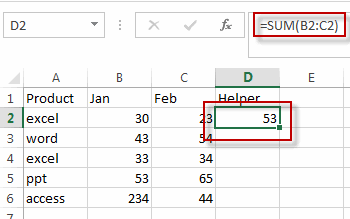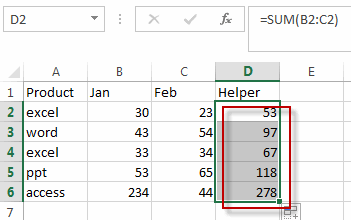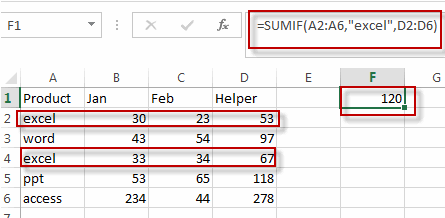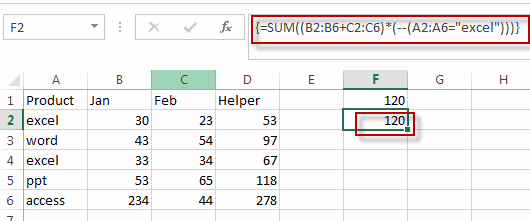# Sum Multiple Columns based on One Criteria

This post will guide you how to sum multiple columns based on the same criteria in Excel. How do I sum multiple columns based on single criteria in Excel. How to sum multiple columns using one single condition with a formula in Excel.

## Sum Multiple Columns based on One Criteria

Assuming that you have a list of data that contain product names in column A, and have sales values for the first two month in Column B and C. and you want to sum all sale values for product “excel” in all two months. How to achieve it. You need to create a formula based on the SUM function to sum sale values for all two months in a newly helper column. And then create another formula based on the SUMIF function to sum total values for product “excel”. Do the following steps:

#1 Type the following formula in a helper column, and then press Enter key to apply this formula.

`=SUM(B2:C2)`#2 drag the AutoFill Handle over other cells to apply this formula. And the total of sale values in first two months are calculated in the helper column.#3 type the following into a blank cell and then press Enter key in your keyboard.

`=SUMIF(A2:A6,"excel",D2:D6)`You can also use an Excel array formula based on the SUMIF function to achieve the same result. Like this:

`=SUM((B2:B6+C2:C6)*(--(A2:A6="excel")))`

Type this formula into a blank cell, and then press ctrl+Shift+Enter shortcut keys to apply this array formula in Excel.

Let’s see the result:### Related Functions

• Excel SUM function
The Excel SUM function will adds all numbers in a range of cells and returns the sum of these values. You can add individual values, cell references or ranges in excel.The syntax of the SUM function is as below:= SUM(number1,[number2],…)…
• Excel SUMIF Function
The Excel SUMIF function sum the numbers in the range of cells that meet a single criteria that you specify. The syntax of the SUMIF function is as below:=SUMIF (range, criteria, [sum_range])…